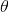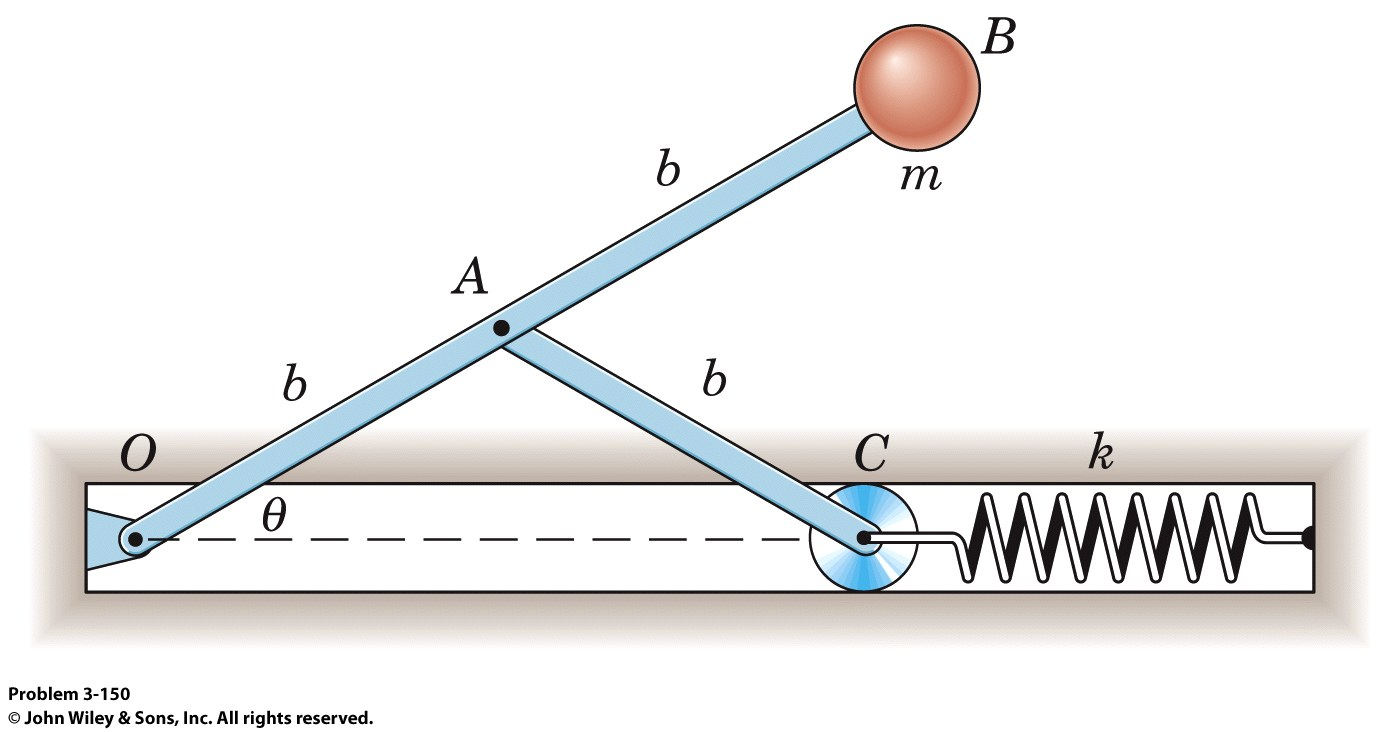### Create an Account

Home / Questions / The 08 kg particle is attached to the system of two light rigid bars all of which move in ...

# The 08 kg particle is attached to the system of two light rigid bars all of which move in a vertical plane The spring is compressed an amount b2 when theta 0 and the length b 030 m The

The 0.8-kg particle is attached to the system of two light rigid bars, all of which move in a vertical plane. The spring is compressed an amount b/2 when= 0, and the length b = 0.30 m. The system is released from rest in a position slightly above that for= 0. (a) if the maximum value ofis observed to be 50o, determine spring constant k. (b) For k = 400 N/m, determine the speed v of the particle when= 25o. Also find the corresponding value of d/dt.Subscribe To Get Solution